American Journal of Modern Physics
Volume 4, Issue 6, November 2015, Pages: 287-290

Impurity Binding Energyin Quantum Dots with Parabolic Confinement in the Presence of Electric Field

Arnold Abramov1, Zhiya Zhao1, Alexander Kostikov2

1Kuang-Chi Institute of Advance Technology, Shenzhen, China

2Donbass State Engineering Academy, Kramatorsk, Ukraine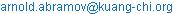(A. Abramov)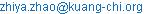(Z. Zhao)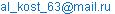(A. Kostikov)

Arnold Abramov, Zhiya Zhao, Alexander Kostikov. Impurity Binding Energyin Quantum Dots with Parabolic Confinement in the Presence of Electric Field. American Journal of Modern Physics. Vol. 4, No. 6, 2015, pp. 287-290. doi: 10.11648/j.ajmp.20150406.15

Abstract: We present an efficient method for calculation of the impurity binding energy in a quantum dot with parabolic confinement in the presence of the electric field. The unknown wave function is expanded into a basis of one-dimensional harmonic oscillator states describing the electron's movement perpendicular to the plane of quantum well. Green's function technique used to calculate the coefficients of the expansion. Binding energy of impurity states is defined as poles of the wave function. Developed method applied to calculation of impurity binding energy for different position of impurity and the intensity of electric field.

Keywords: Quantum Dot, Electric Field, Impurity

Contents

1. Introduction

Quantum dots are artificial structures in which the motion of the charge carriers is limited in three dimensions. The type of confinement potential used determines their possible states, such as the band structure and the basic physical properties of quantum dots (QD). Two important factors are the impurity states (IS) and the external field, without which it would be difficult to practically implement QDs in electronics and they can affect the band structure. Since Bastard calculated  for the very first time the impurity binding energy in QW many works on this subject have been published. Over the last three decades, new calculation methods have been developed to determine the binding energy of IS in QWs and QDs, by assuming the form of confinement potential [2-5] and the features of the band structure [5-9]. The form of the confinement potential is a significant characteristic. In particularly, (as mentioned in ref. ) advantages of devices based on QDs (compared with QWs) has become possible because of the synthesis of QDs that satisfy the rigid requirements of size, shape, uniformity, and density which ultimately affect the choice of the potential used to confine the charge carriers in QDs. Although the most widely used model for QDs has a spherical symmetry potential, allowing for simple and convenient (for the following analysis) solutions, it only provides a qualitative pictures, because there are many factors that reduce the symmetry, making the problem very complicated. In this sense, a model with a parabolic confinement and a low symmetry is more adequate. The practicality of this model has been demonstrated in many studies (see  and the references therein). A study of the effect of an external field on the energy spectrum of low dimensional structures also led to the work performed by Bastard , where the electron eigenstates in a QW under an electric field were calculated.

Electric field is additional tool which can affect the band structure of low dimensional sample. Recently effects of an electric field on the binding energy of impurity states in QD's have been reported in [12-17].

In the present study the problem of the impurity binding in QDs with parabolic confinement, under an electric field is considered and solved. The approach did not use any additional (non physical) parameters, allowing for the control of the accuracy of the results obtained.

2. Theoretical Background

Within the framework of effective-mass approximation, the Hamiltonian of an electron bound to donor impurity in a spherical QD with parabolic confinement in the presence of electric field along the z-axis is given as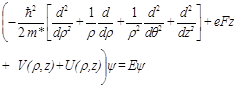(1)

where cylindrical coordinates (r, q) are the distance and angle describing the position of an electron in the plane perpendicular to the electric field; F is external electric field applied in the z-direction; m* is the effective mass of the electron, V(r,z)=1/2m*w2(r2+z2), w - angular frequency of the parabolic confinement potential,- Coulomb interaction between an electron and the impurity ion, e – unit charge, k - permittivity, k0 - dielectric constant, z0 – impurity position. Because of the axial symmetry of the system, angular momentum projection onto the z axis is conserved Lz=h m (m=0, 1, 2, …- magnetic quantum number), and their eigenfunctions exp(imz) determine the dependence of the unknown electron wave function versus an angle: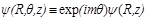. By solving the one-dimensional Schrödinger equation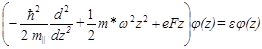(2)

we get the basis for an expansion of the WF: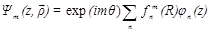(3)

Farther we use the next units for distance (Bohr radius ab), energy (Rydberg Ry) and electric field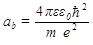,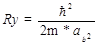,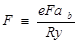and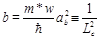measure the strength of the confinement potential. Also we input here parameter Lc corresponding to the dimension of the QD . Solutions of equation (2) describe one dimensional harmonic oscillator states: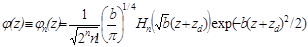,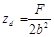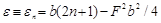(4)

where Hn - Hermitepolynom of n-th order.

Substituting (3) in (1), we obtain a system of differential equations.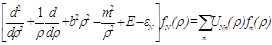(5)

where.

To construct the solutions of (5) we introduce the Green's function GN (r,r') via the equation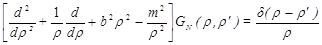Thus the expression for fN(r) can be written as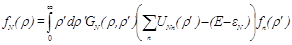(6)

The expression for Green's function GN(r,r'), as well as method of solution for equation (6) is given in [17, 18]. The solution of the equation (6) allows finding the impurity binding energy for various impurity location and values of the intensity of electric field.

3. Results and Discussion

The results of calculation for impurity binding energyas function of electric field are shown on Fig. 1. These results are in qualitative and quantitativeagreement with last published data . The common feature of the two dependencies is the decrease of binding energy due to the increase of the electric field. Note that these dependencies are for the case when the impurity atom is placed in the center of QD. As electric field increase the maximum of the electron wave function is shifted from the center to the distance zd (see (4)). Thus, the average distance between the electron and impurity ion is increased, and the binding energy, respectively, decreases.

We note also that the relative change of the binding energy for range of electric field 0-300 kV/cm is decrease as confinement potential of QD is increase. It occurs because value of zd will be smaller for large values of the potential. Accordingly, for confinement potential of strength hw=100 mev the average distance between impurity ion and electron will be less than for hw=50 mev. Thus, as we can see from Fig. 1 relative change of the binding energy is smaller for the case of hw=100 mev.

Now we consider Fig. 2. It presents binding energy as a function of the impurity location, for values of the electric field of 0 and 250 kV/cm and the value of confinement potential hw=100 meV. The value F=250 kV/cm for electric field is chosen so that it corresponds to the case when zd is very close to Lc (zd=0.29, Lc=0.34). In the case of the zero electric field the movement of the impurity atom from the centre to the edge led to the increase of the average distance between impurity ion and the electron (maximum of electron WF remains in the center). Therefore, the binding energy will decrease. In the presence of electric field F=250 kV/cm the maximum of WF are at the edge of the QD, and therefore, if z0 = -1 binding energy have maximal value, and decreases with the displacement of the impurity atom from one side to the other.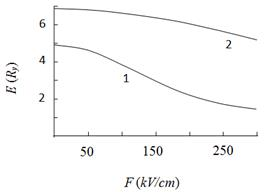Fig. 1. Binding energy as function of the electric field for different strength of confinement potential, hw (mev): 1 - 50; 2-100.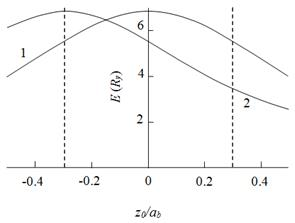Fig. 2. Binding energy as function of the impurity position.Electric field (kV/cm): 1 - 0; 2- 250. The dashed lines correspond to QD's boundaries.

4. Conclusion

In conclusion, we have calculated thebinding energyin QD with parabolic confinement in the presence of electric field. The electric field shifts the maximum of the electron wave function, thereby changing the average distance between the electron and impurity ion, and as a consequence, the binding energy. It is shown that effect of electric field on the binding energy is equivalent to the change of impurity location. This result is of practical value, allowing changing the binding energy avoiding application of electric fields.

The work was supported by Guangdong Innovative Research Team Program (No. 2009010005), Shenzhen Innovative R&D Team Program (Peacock Plan) (No. KQE201106020031A), Shenzhen Key Laboratory of Optical and Terahertz Meta-RF (No. CXB201109210101A).

References

1. G. Bastard, "Hydrogenic impurity states in a quantum well: a simple model", Phys. Rev. B,vol. 24 pp.4714-4722, 1981.
2. C.Y.Ngo, S.F.Yoon, and W.J.Fan, S.J.Chua, "Effects of size and shape on electronic states of quantum dots", Phys.Rev.B,vol. 74,pp. 245331, 2006.
3. Y. Turki-Ben Alia, G. Bastard, R. Bennaceur, "Ground state transition energies in biased InAs/GaAs quantum dots",Physica E,vol. 27, pp. 67-76, 2005.
4. M. Willatzen, L.C. Lew Yan Voon, "Confined states in parabolic cylinder quantum dots",Physica E,vol. 16,pp. 286-296, 2003.
5. S.M.Reimann, M.Manninen, "Electronic structure of quantum dots", Rev. of Mod. Phys.,vol. 74,pp.1283-1342, 2002.
6. Y. Hada, M. Eto, "Electronic states in silicon quantum dot devices", Phys. stat. sol. (c),vol. 2, pp.3035-3038, (2005).
7. F. B. Pedersen, Yia-Chung Chang,"Magnetic-field effects on one- and two-hole states in parabolic quantum dots", Phys. Rev. B, vol. 55,pp. 4580-4588, 1997.
8. C.Pryor,"Eight-band calculations of strained InAs/GaAs quantum dots compared with one-, four-, and six-band approximations", Phys. Rev. B, vol. 57, pp. 7190-7196,1998.
9. Curt A. Flory Charles B. "Musgrave and Zhiyong Zhang, Quantum dot properties in the multiband envelope-functionapproximation using boundary conditions based upon first-principles quantum calculations", Phys. Rev. B, vol. 77, pp. 205312, 2008.
10. N. N. Ledentsov, V.M. Ustinov, V.A. Shchukin. P.S. Kop’ev, and Zh.I. Alferov, "Quantum dot heterostructures: fabrication, properties, lasers (Review)", Semiconductors, vol. 32, pp.343-365, 1998.
11. G. Bastard, E. E. Mendez, L. L. Chang, L. Esaki, "Variational calculations on a quantum well in an electric field", Phys. Rev. B, vol. 28, pp. 3241-3245, 1983.
12. Jian-Hui Yuan, Yan Zhang, Meng Li, Zhi-Hui Wu, Hua Mo, "Hydrogenic impurity states in a parabolic quantum dot: Hydrostatic pressure and electricfield effects", Superlattices and Microstructures, vol. 74, pp. 1-10, (2014).
13. P. Jiang-Hong, L. Li-Zhe, L. Min, "Hydrogenic-Donor Impurity States in GaAs/AlxGa1−xAs Quantum Dots in thePresence of an Electric Field", Chin. Phys. Lett.,vol. 28, pp. 086201, 2011.
14. M. Tshipa, "The effect of confining electric potentials on binding energiesin a spheroidal quantum dot", Indian J. Phys.,vol. 86, pp. 807-812, 2012.
15. A. John Peter, VemuriLakshminarayana, "Effects of Electric Field on Electronic States in a GaAs/GaAlAs Quantum Dotwith Different Confinements", Chin. Phys. Lett.,vol. 25,pp. 3021-3024, 2008.
16. J.-H. Yuan, Y. Zhang, X. Guo, J. Zhang, H.Mo, "The low-lying states and optical absorption properties of a hydrogenic impurity in a parabolic quantum dot modulation by applied electric field", PhysicaE, vol. 68, pp. 232–238, 2015.
17. S. Wang, Y. Kang, X.-L. Li, "Binding energy of the ground and first few excited states of hydrogenic donor impurity in a rectangular GaAs quantum dot in the presence of electric field", Superlattices and Microstructures, vol. 76, pp.221–233, 2014.
18. N.V.Lien, N.M.Trinh, "Electric field effects on the binding energy of hydrogen impurities in quantum dots with parabolic confinements", J. Phys.: Condens. Matter, vol. 13, pp. 2563–2571, 2001.
19. A. A. Abramov, "Resonant donor states in quantum well", Mod. Phys. Lett. B, vol. 25, pp. 89-96, 2011.
20. A. A. Abramov, "Impurity binding energies in quantum dots with parabolic confinement", Physica E, vol. 67, pp. 28-32, 2015.

 Contents 1. 2. 3. 4.
Article ToolsAbstractPDF(307K)